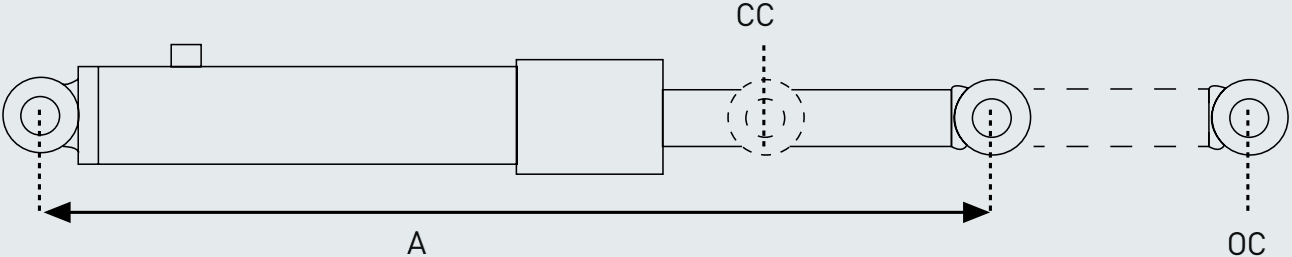# Calculator### These formulas are for your guide only -for more indepth calculations please consult your registered design engineer.

mm
inches

P - maximum safe load (tonnes) = tonnes

### Oil volume calculator

Cylinder effective area -
Cylinder volume capacity (extended) -
Cylinder volume capacity (retracted) -

Push -
Pull -

### Stroke & closed centres given the open centresTip: use either mm or inches for this calculator and the result will be in the same unit (do not mix units).

A = Open centre

B = Dead length = + + + + + =

Stroke = ( -B )/2=

Closed centre = stroke+B= (minimum possible, can be more)

### Open & closed centres given the mid positionTip: use either mm or inches for this calculator and the result will be in the same unit (do not mix units).

A = Mid position A

B = + + + + + =

Stroke = ( -B )÷1.5=

Closed centre = stroke+B=

Open centre=closed centre+stroke=